# NCERT Solutions For Class 10 Maths Chapter 11 Exercise 11.2 Constructions

Download NCERT Solutions For Class 10 Maths Chapter 11 Exercise 11.2 Constructions. This Exercise contains 7 questions, for which detailed answers have been provided in this note. In case you are looking at studying the remaining Exercise for Class 10 for Maths NCERT solutions for other Chapters, you can click the link at the end of this Note.

### NCERT Solutions For Class 10 Maths Chapter 11 Exercise 11.2 Constructions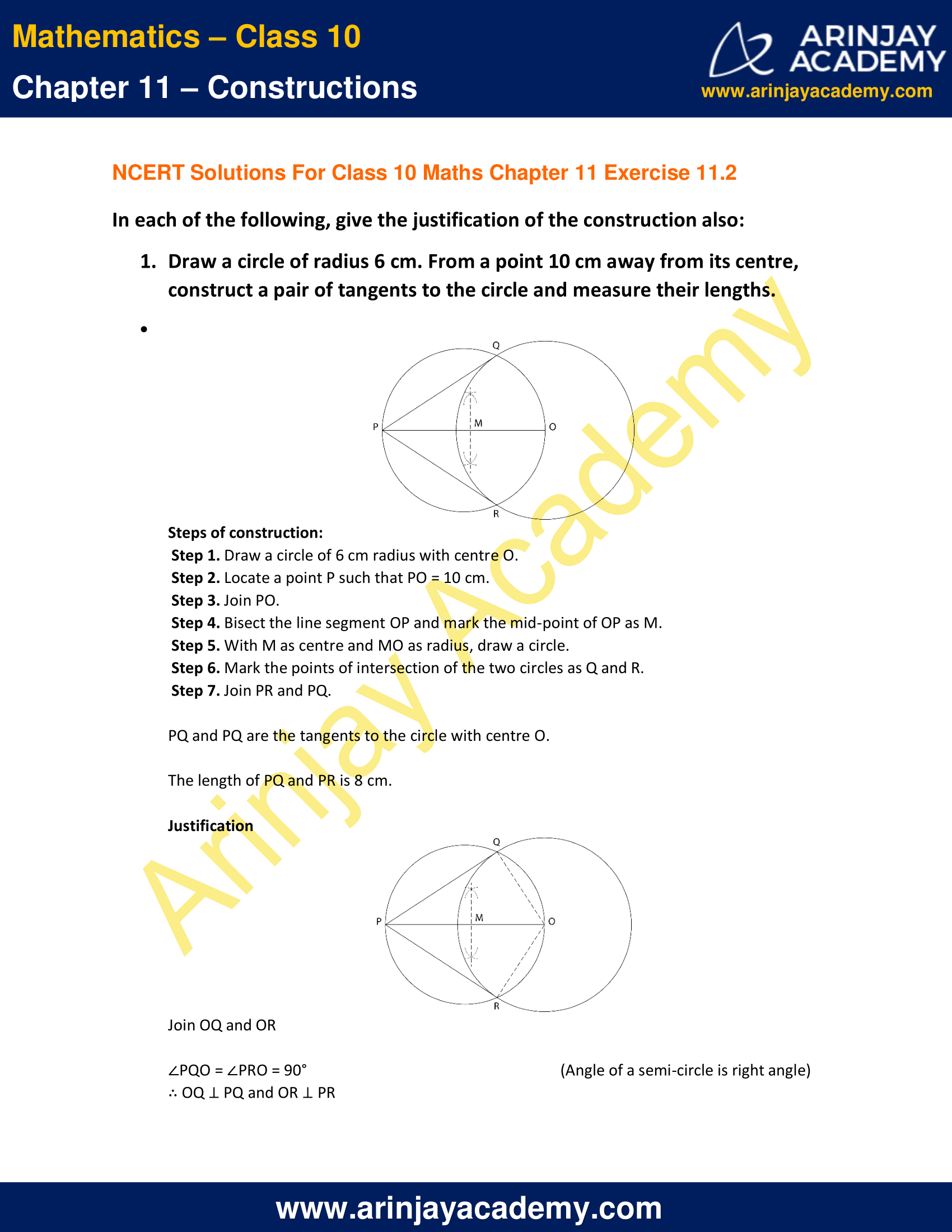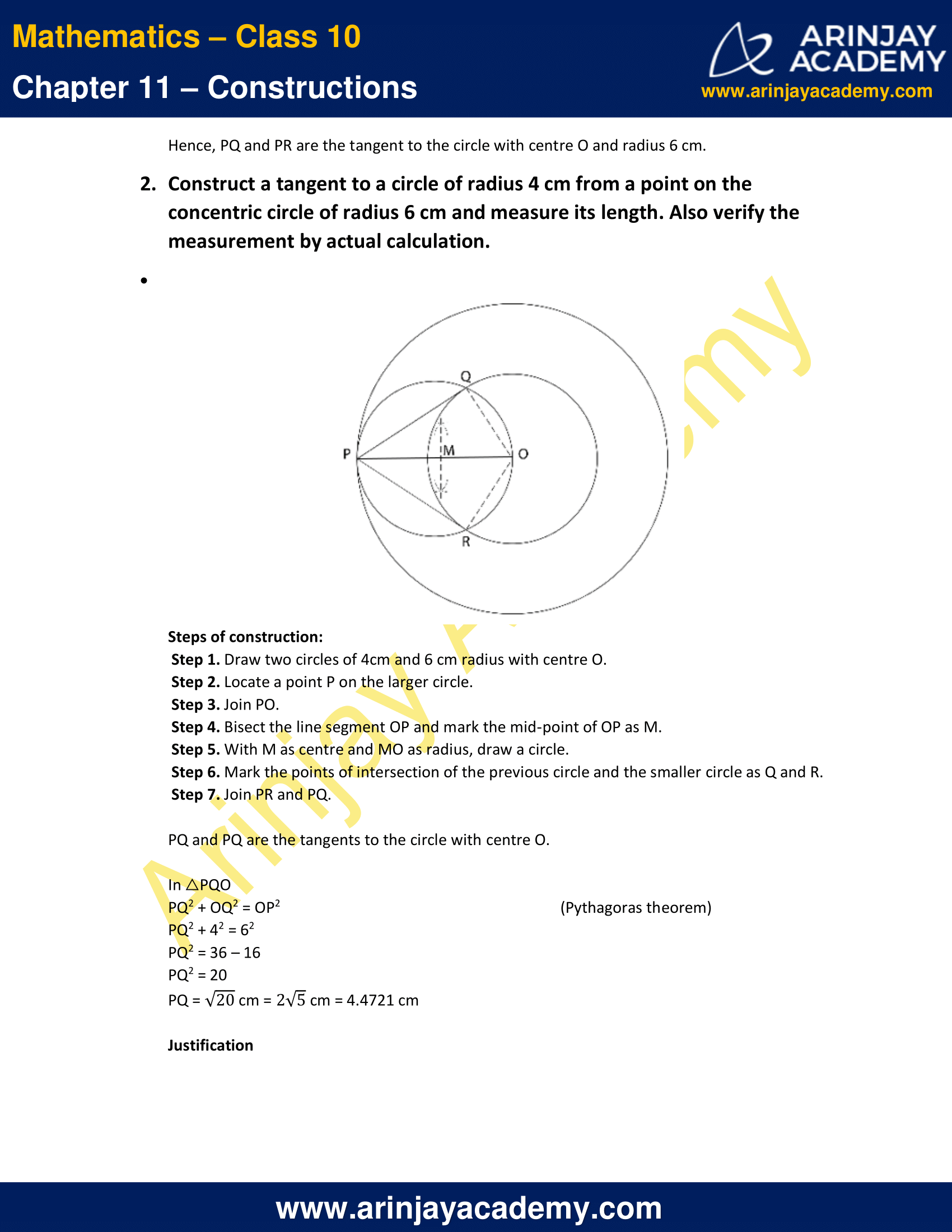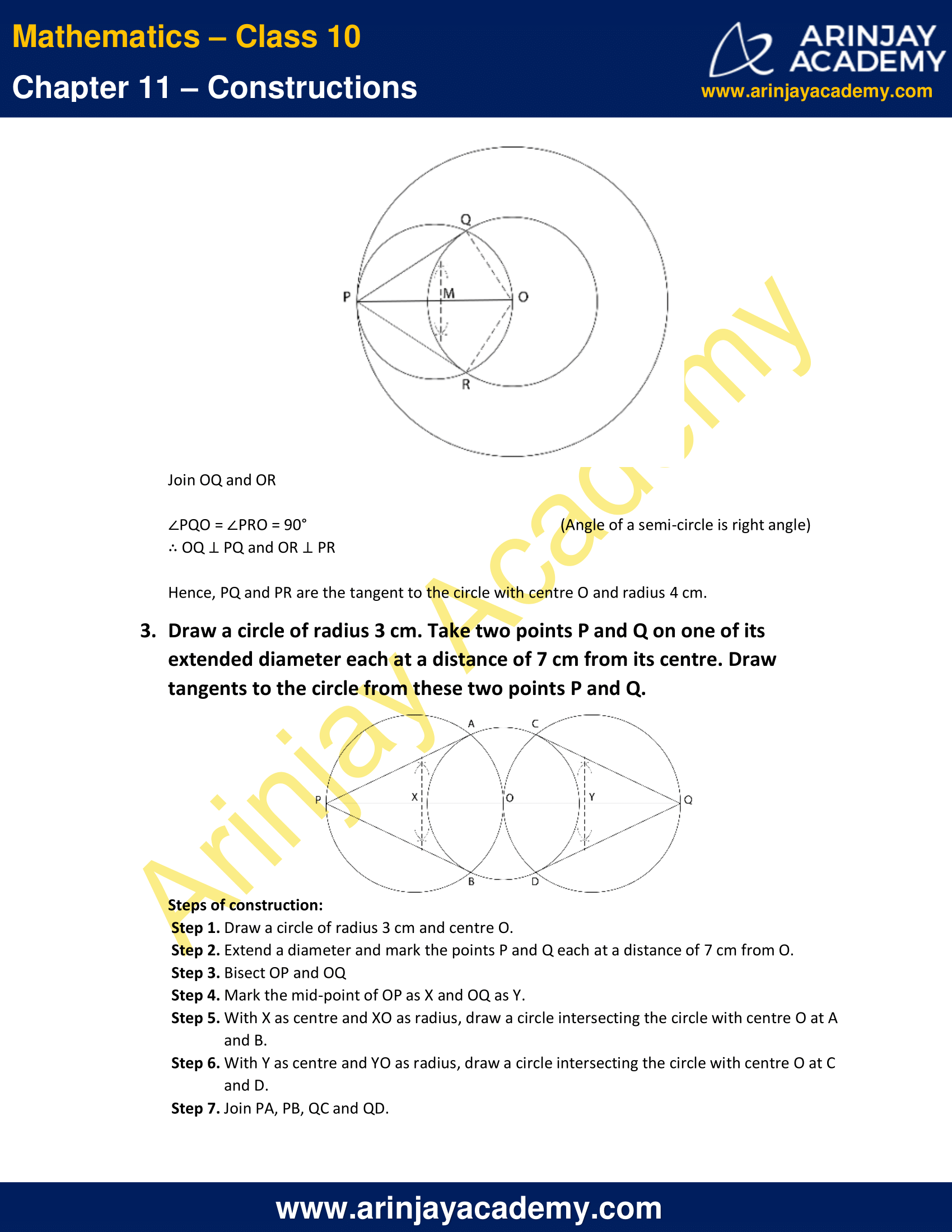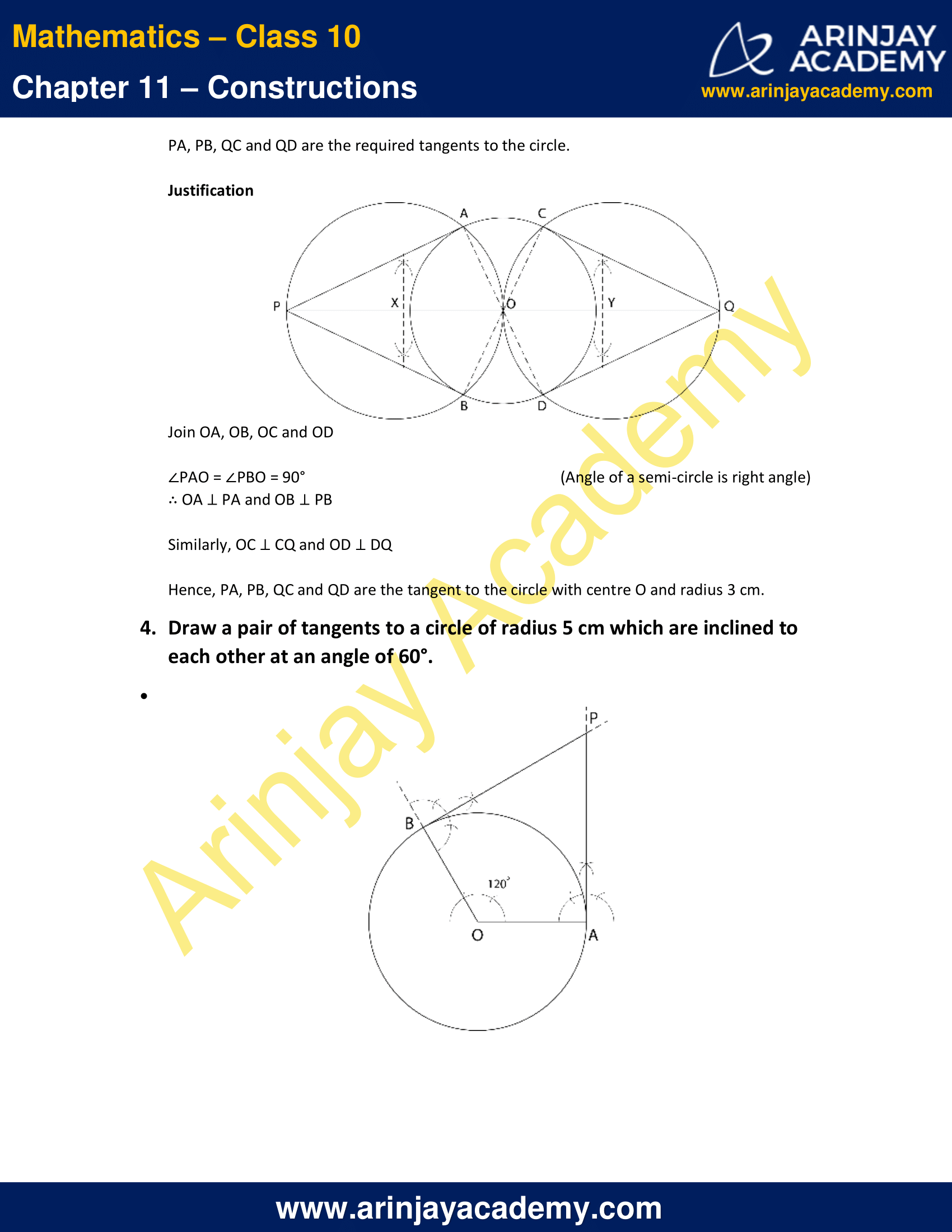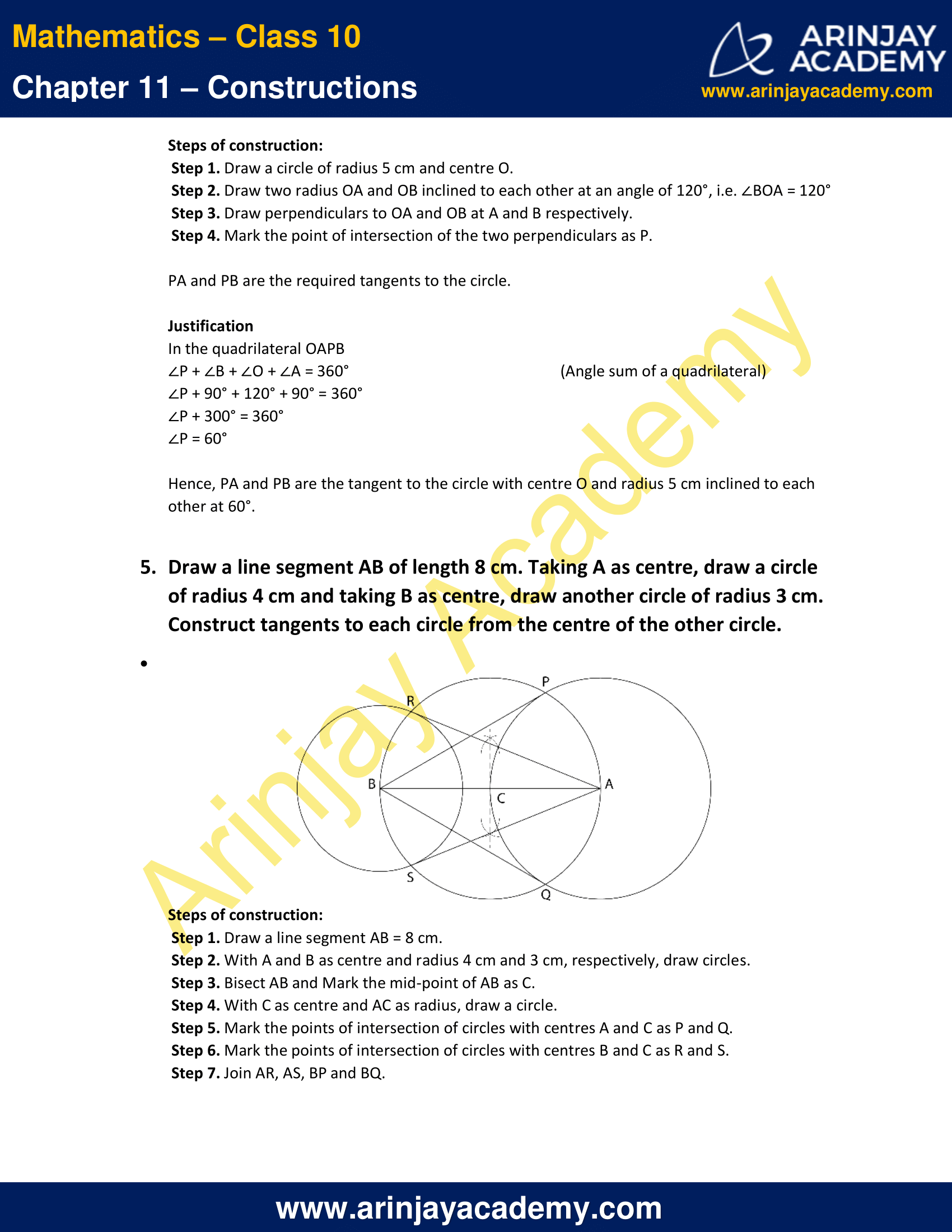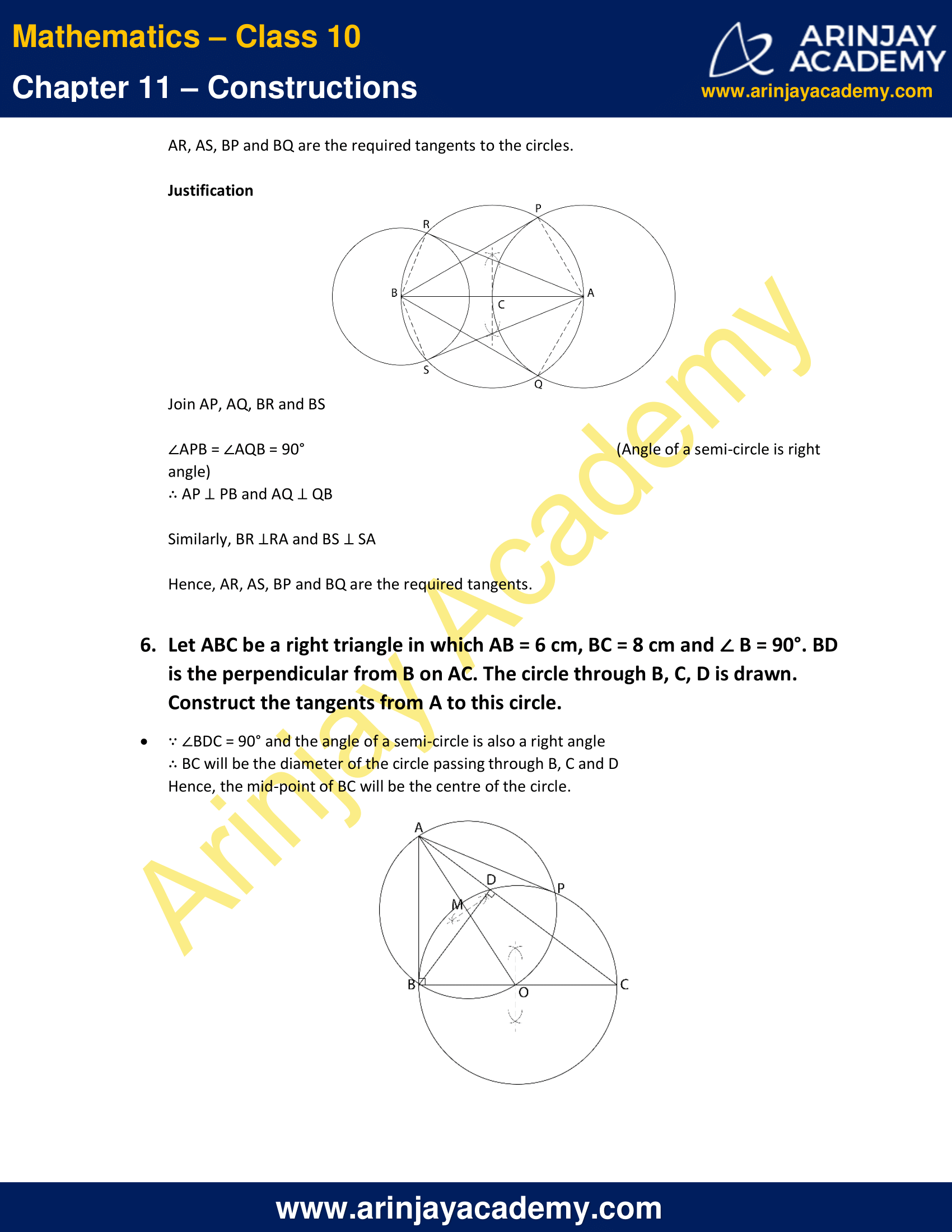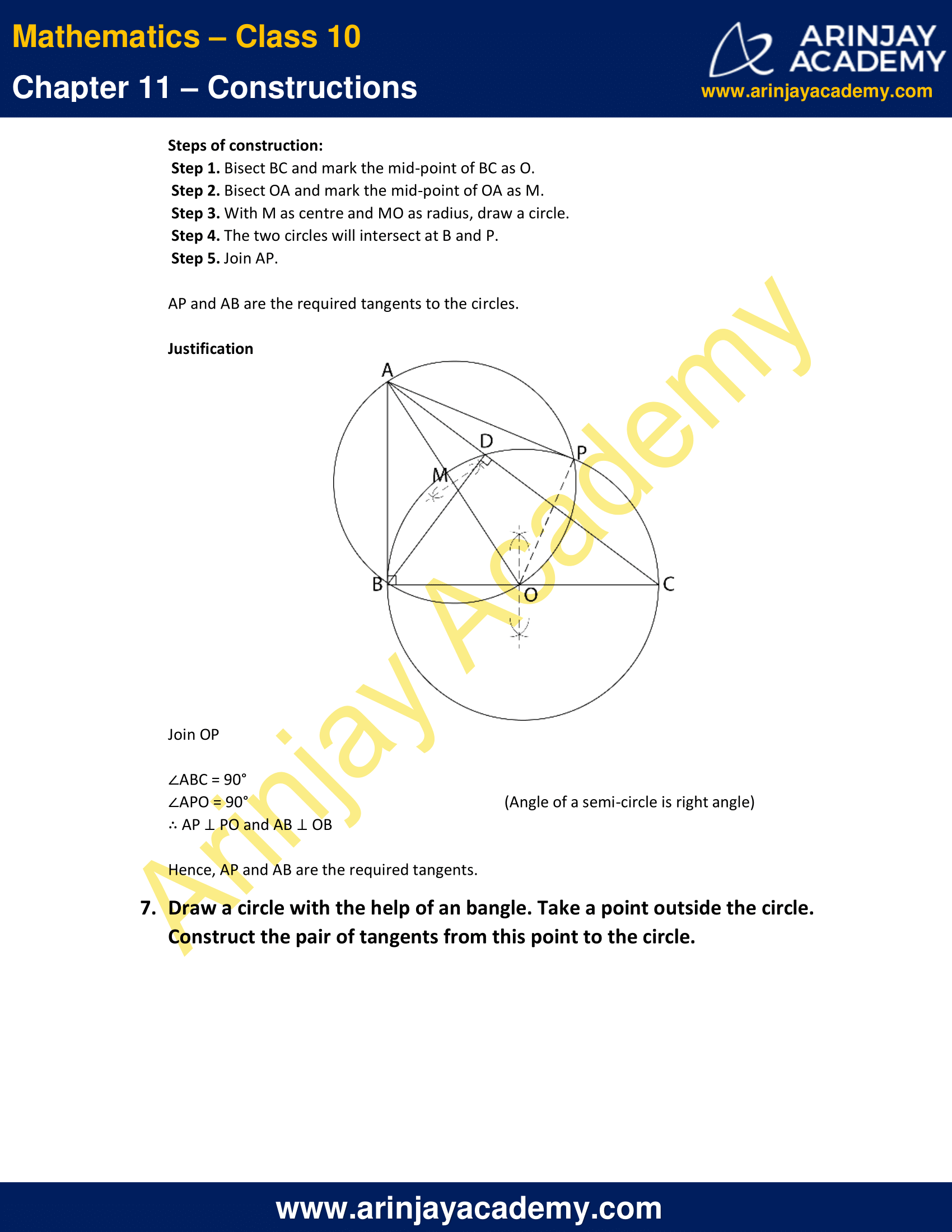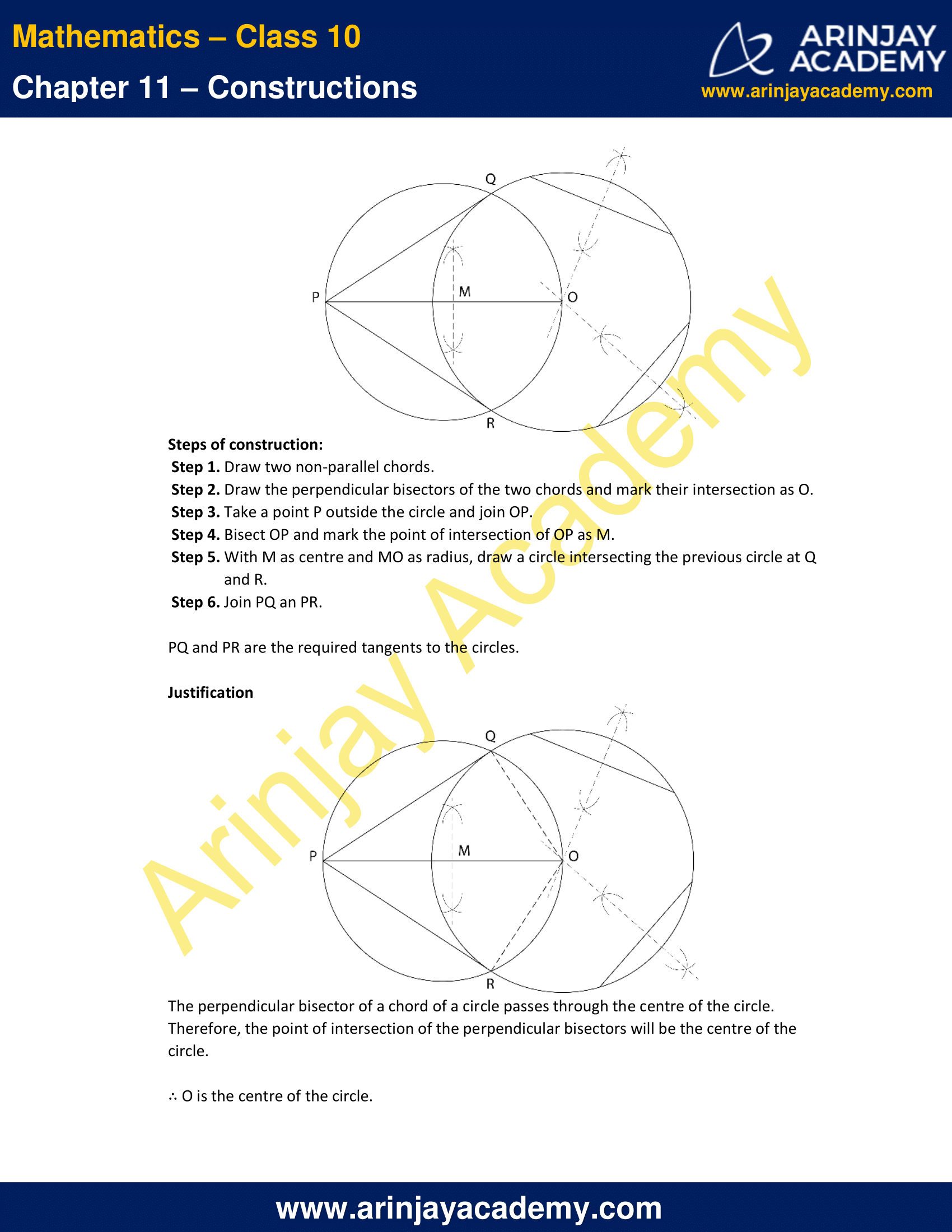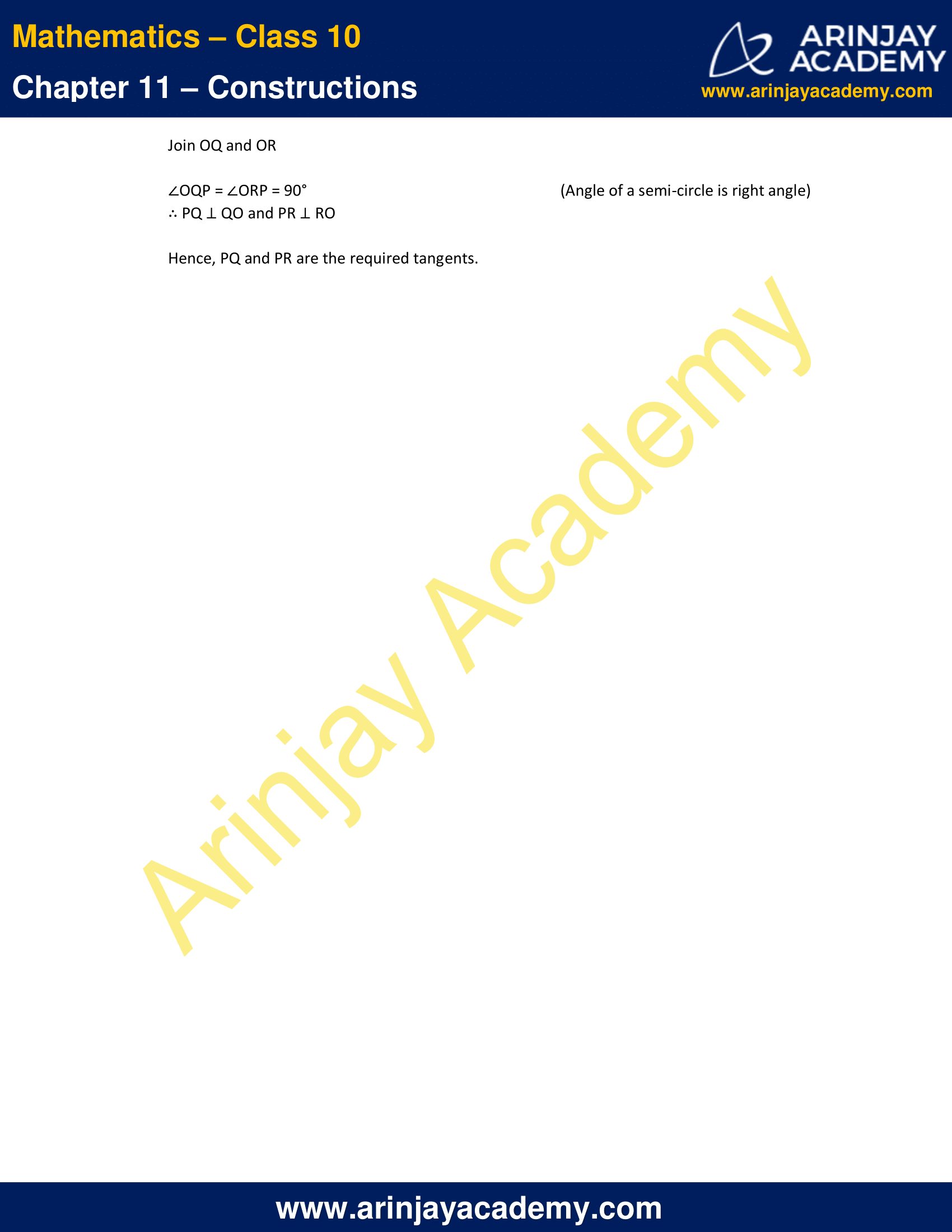NCERT Solutions For Class 10 Maths Chapter 11 Exercise 11.2 Constructions

In each of the following, give the justification of the construction also:

1. Draw a circle of radius 6 cm. From a point 10 cm away from its centre, construct a pair of tangents to the circle and measure their lengths.

Solution:Steps of construction:

• Step 1. Draw a circle of 6 cm radius with centre O.
• Step 2. Locate a point P such that PO = 10 cm.
• Step 3. Join PO.
• Step 4. Bisect the line segment OP and mark the mid-point of OP as M.
• Step 5. With M as centre and MO as radius, draw a circle.
• Step 6. Mark the points of intersection of the two circles as Q and R.
• Step 7. Join PR and PQ.

PQ and PQ are the tangents to the circle with centre O.
The length of PQ and PR is 8 cm.

JustificationJoin OQ and OR
∠PQO = ∠PRO = 90°  (Angle of a semi-circle is right angle)
∴ OQ ⊥ PQ and OR ⊥ PR
Hence, PQ and PR are the tangent to the circle with centre O and radius 6 cm.

2. Construct a tangent to a circle of radius 4 cm from a point on the concentric circle of radius 6 cm and measure its length. Also verify the measurement by actual calculation.

Solution: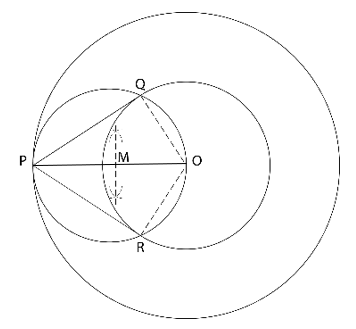Steps of construction:

• Step 1. Draw two circles of 4cm and 6 cm radius with centre O.
• Step 2. Locate a point P on the larger circle.
• Step 3. Join PO.
• Step 4. Bisect the line segment OP and mark the mid-point of OP as M.
• Step 5. With M as centre and MO as radius, draw a circle.
• Step 6. Mark the points of intersection of the previous circle and the smaller circle as Q and R.
• Step 7. Join PR and PQ.

PQ and PQ are the tangents to the circle with centre O.

In △PQO
PQ2 + OQ2 = OP2  (Pythagoras theorem)
PQ2 + 42 = 62
PQ2 = 36 – 16
PQ2 = 20
PQ = √20  cm = 2√5 cm = 4.4721 cm

JustificationJoin OQ and OR
∠PQO = ∠PRO = 90° (Angle of a semi-circle is right angle)
∴ OQ ⊥ PQ and OR ⊥ PR

Hence, PQ and PR are the tangent to the circle with centre O and radius 4 cm.

3. Draw a circle of radius 3 cm. Take two points P and Q on one of its extended diameter each at a distance of 7 cm from its centre. Draw tangents to the circle from these two points P and Q.

Solution:Steps of construction:

• Step 1. Draw a circle of radius 3 cm and centre O.
• Step 2. Extend a diameter and mark the points P and Q each at a distance of 7 cm from O.
• Step 3. Bisect OP and OQ
• Step 4. Mark the mid-point of OP as X and OQ as Y.
• Step 5. With X as centre and XO as radius, draw a circle intersecting the circle with centre O at A and B.
• Step 6. With Y as centre and YO as radius, draw a circle intersecting the circle with centre O at C and D.
• Step 7. Join PA, PB, QC and QD.

PA, PB, QC and QD are the required tangents to the circle.

JustificationJoin OA, OB, OC and OD
∠PAO = ∠PBO = 90°   (Angle of a semi-circle is right angle)
∴ OA ⊥ PA and OB ⊥ PB

Similarly, OC ⊥ CQ and OD ⊥ DQ
Hence, PA, PB, QC and QD are the tangent to the circle with centre O and radius 3 cm.

4. Draw a pair of tangents to a circle of radius 5 cm which are inclined to each other at an angle of 60°.

Solution: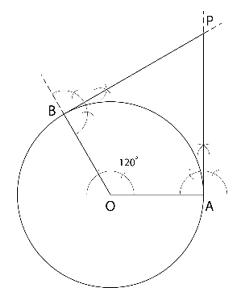Steps of construction:

• Step 1. Draw a circle of radius 5 cm and centre O.
• Step 2. Draw two radius OA and OB inclined to each other at an angle of 120°, i.e. ∠BOA = 120°
• Step 3. Draw perpendiculars to OA and OB at A and B respectively.
• Step 4. Mark the point of intersection of the two perpendiculars as P.

PA and PB are the required tangents to the circle.

Justification

In the quadrilateral OAPB
∠P + ∠B + ∠O + ∠A = 360°  (Angle sum of a quadrilateral)
∠P + 90° + 120° + 90° = 360°
∠P + 300° = 360°
∠P = 60°

Hence, PA and PB are the tangent to the circle with centre O and radius 5 cm inclined to each other at 60°.

5. Draw a line segment AB of length 8 cm. Taking A as centre, draw a circle of radius 4 cm and taking B as centre, draw another circle of radius 3 cm. Construct tangents to each circle from the centre of the other circle.

Solution: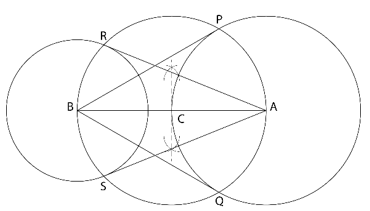Steps of construction:

• Step 1. Draw a line segment AB = 8 cm.
• Step 2. With A and B as centre and radius 4 cm and 3 cm, respectively, draw circles.
• Step 3. Bisect AB and Mark the mid-point of AB as C.
• Step 4. With C as centre and AC as radius, draw a circle.
• Step 5. Mark the points of intersection of circles with centres A and C as P and Q.
• Step 6. Mark the points of intersection of circles with centres B and C as R and S.
• Step 7. Join AR, AS, BP and BQ.

AR, AS, BP and BQ are the required tangents to the circles.

Justification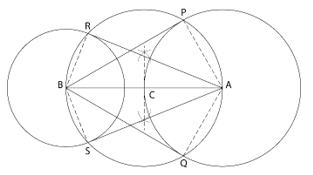Join AP, AQ, BR and BS
∠APB = ∠AQB = 90°  (Angle of a semi-circle is right angle)
∴ AP ⊥ PB and AQ ⊥ QB
Similarly, BR ⊥RA and BS ⊥ SA
Hence, AR, AS, BP and BQ are the required tangents.

6. Let ABC be a right triangle in which AB = 6 cm, BC = 8 cm and ∠ B = 90°. BD is the perpendicular from B on AC. The circle through B, C, D is drawn. Construct the tangents from A to this circle.

Solution:

∵ ∠BDC = 90° and the angle of a semi-circle is also a right angle
∴ BC will be the diameter of the circle passing through B, C and D
Hence, the mid-point of BC will be the centre of the circle.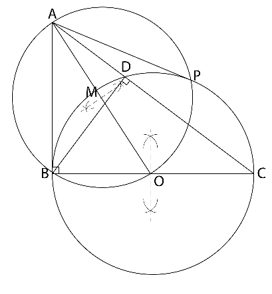Steps of construction:

• Step 1. Bisect BC and mark the mid-point of BC as O.
• Step 2. Bisect OA and mark the mid-point of OA as M.
• Step 3. With M as centre and MO as radius, draw a circle.
• Step 4. The two circles will intersect at B and P.
• Step 5. Join AP.

AP and AB are the required tangents to the circles.

Justification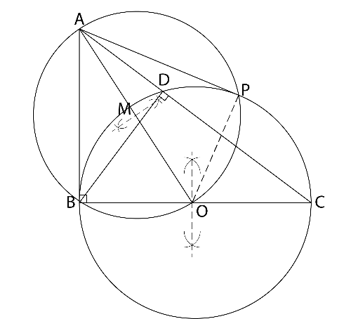Join OP
∠ABC = 90°
∠APO = 90°  (Angle of a semi-circle is right angle)
∴ AP ⊥ PO and AB ⊥ OB
Hence, AP and AB are the required tangents.

7. Draw a circle with the help of an bangle. Take a point outside the circle. Construct the pair of tangents from this point to the circle.

Solution: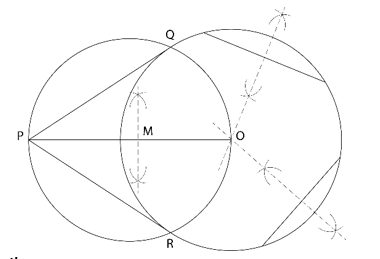Steps of construction:

• Step 1. Draw two non-parallel chords.
• Step 2. Draw the perpendicular bisectors of the two chords and mark their intersection as O.
• Step 3. Take a point P outside the circle and join OP.
• Step 4. Bisect OP and mark the point of intersection of OP as M.
• Step 5. With M as centre and MO as radius, draw a circle intersecting the previous circle at Q and R.
• Step 6. Join PQ an PR.

PQ and PR are the required tangents to the circles.

Justification

The perpendicular bisector of a chord of a circle passes through the centre of the circle. Therefore, the point of intersection of the perpendicular bisectors will be the centre of the circle.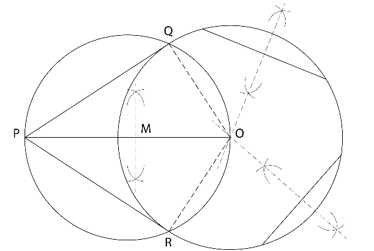∴ O is the centre of the circle.
Join OQ and OR
∠OQP = ∠ORP = 90°  (Angle of a semi-circle is right angle)
∴ PQ ⊥ QO and PR ⊥ RO
Hence, PQ and PR are the required tangents.

NCERT Solutions for Class 10 Maths Chapter 11 Exercise 11.2 Constructions, has been designed by the NCERT to test the knowledge of the student on the topic – Construction of Tangents to a Circle

Download NCERT Solutions For Class 10 Maths Chapter 11 Exercise 11.2 Constructions